• 2022-05-02 21:01:58

## Python合并多个sheet工作表为一个工作表

这里以四级单词为例，pdf转换为表格后，多达113个sheet。
话不多说，直接上代码，没有相应的库。

注意：运行程序前不能打开该工作表

pip install 相应库名

import xlrd
import pandas as pd
from pandas import DataFrame

excel_name = r"D:\四级词汇.xlsx"
wb = xlrd.open_workbook(excel_name)
sheets = wb.sheet_names()

alldata = DataFrame()
for i in range(len(sheets)):
df = pd.read_excel(excel_name, sheet_name=i, index=False, encoding='utf8')
alldata = alldata.append(df)

writer = pd.ExcelWriter(r"D:\四级词汇.xlsx", engine='openpyxl')
writer.book = book
# 必须要有上面这两行，假如没有这两行，则会删去其余的sheet表，只保留最终合并的sheet表，合并的sheet表在最后一个，名为“ALLDATA ”
alldata.to_excel(excel_writer=writer, sheet_name="ALLDATA")
writer.save()
writer.close()
print("完成,请到文件夹查看")



如果出现错误如：
PermissionError: [Errno 13] Permission denied: ‘D:\APP\ivx\四级词汇.xlsx’
表示该工作表已经打开，不能读写，关闭重新运行程序即可。

完成效果：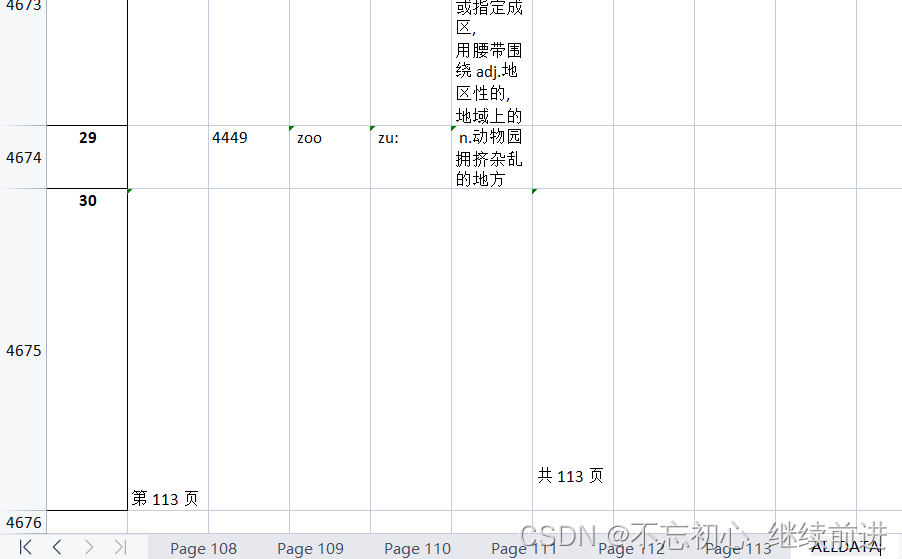开发语言
更多相关内容
• 主要介绍了解决python pandas读取excel中多个不同sheet表格存在的问题，具有很好的参考价值，希望对大家有所帮助。一起跟随小编过来看看吧
• 一个表格中有多个sheet文件 代码： import pandas as pd #读取excel所有sheet数据 df=pd.read_excel('BI练习题(1).xlsx', sheet_name=None) # 输出各个sheet名 print(df.keys()) # 输出该表格内容 print(df['产品...

说明
一个表格中有多个sheet文件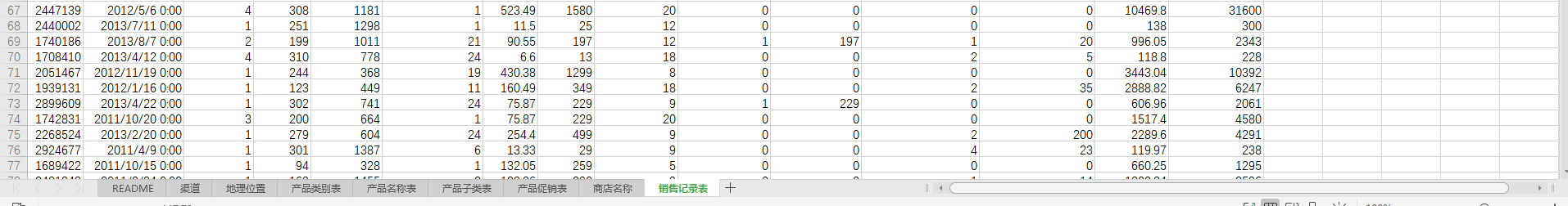代码：

import pandas as pd
#读取excel所有sheet数据
# 输出各个sheet名
print(df.keys())
# 输出该表格内容
print(df['产品类别表'])


结果：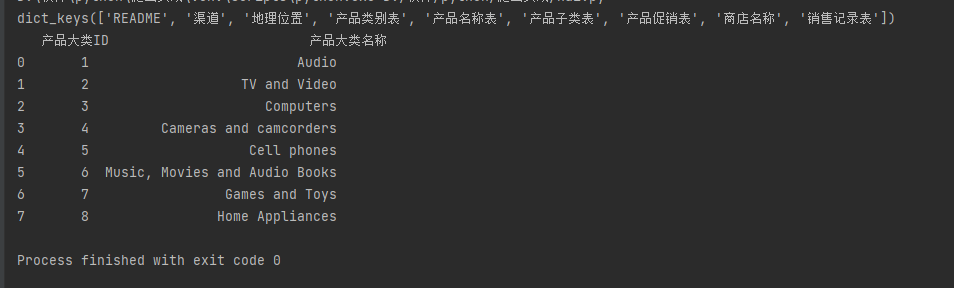展开全文• 将一列表数据写入output.xlsx的a,b,c……等sheet中 import pandas as pd df1 = pd.DataFrame({'a':[3,1],'b':[4,3]}) df2 = df1.copy() with pd.ExcelWriter('F:\\python入门\\数据2\\output.xlsx') as writer: ...
• 之前经常会碰到一个excel有多个sheet文件的情况，当数据少的时候，我们可以把多个sheet文件合成一个xlxs格式的excel，但是当数据很大的时候，我们可以合成一个csv格式的文件，也便于操作。 如图所示，我们可以看到有...

之前经常会碰到一个excel有多个sheet文件的情况，当数据少的时候，我们可以把多个sheet文件合成一个xlxs格式的excel，但是当数据很大的时候，我们可以合成一个csv格式的文件，也便于操作。如图所示，我们可以看到有4个sheet表，现在我们要合并4个sheet表格，但是只保留第一个表格的表头，代码如下：

import csv

import openpyxl

# 获取workbook中所有的表格

sheets = wb.get_sheet_names()

print(sheets)

list1 = []

# 循环遍历所有sheet

for t in range(len(sheets)):

sheet = wb.get_sheet_by_name(sheets[t])

print('\n\n第' + str(t + 1) + '个sheet: ' + sheet.title + '->>>')

#len_row代表表中有多少行数据，len_column代表excel表中有多少列数据

len_row = sheet.max_row

len_column = sheet.max_column

#合并的时候只保留第一张表的表头部分

if t == 0:

for i in range(1,len_row+1):

list2 = []

for j in range(1,len_column+1):

list2.append(sheet.cell(row=i,column=j).value)

list1.append(list2)

else:

for i in range(2,len_row+1):

list2 = []

for j in range(1,len_column+1):

list2.append(sheet.cell(row=i,column=j).value)

list1.append(list2)

#print(list1)

with open('此处填文件路径', 'w', newline='') as f:

writer = csv.writer(f)

writer.writerows(list1)

f.close()

结果：展开全文• 本篇介绍，把多个excel表分别写到一个表格对应的多个sheet里面，每个表的名称就是sheet的名字 import os import pandas as pd dir = './table_dir' # 获取目录下所有的表 origin_file_list = os.listdir(dir) print...

本篇介绍，把多个excel表分别写到一个表格对应的多个sheet里面，每个表的名称就是sheet的名字

#### 第一种方法：用pandas

import os
import pandas as pd

dir = './table_dir'
# 获取目录下所有的表
origin_file_list = os.listdir(dir)
print(origin_file_list)

with pd.ExcelWriter('result.xls') as writer:
# 循环遍历表格
for i in origin_file_list:
# 拼接每个文件的路径
file_path = dir + '/' + i
# 把表名赋予给对应的sheet
sheet_name = i[:-4]

#变相解决表格中第一行第一列为空的缺陷
string = "".join(list(str(i) for i in df.index))
# 判断如果索引都为数字，则不保留索引（根据自己代码调整）
if string.isdigit():
df.to_excel(writer, sheet_name,index=False)
else:
df.to_excel(writer, sheet_name)



#### 第二种方法：用openpyxl

import os
import openpyxl

dir = './table_dir'
# 获取目录下所有的表
origin_file_list = os.listdir(dir)

wb2 = openpyxl.Workbook()
for file in origin_file_list:
file_path = dir + '/' + file
sheet_name = file.split('/')[-1].split('.')
print(sheet_name)
old_sheet_name = old_wb.get_sheet_names()
old_ws = old_wb[old_sheet_name]
ws2 = wb2.create_sheet(sheet_name)
for row in old_ws.values:
ws2.append(row)
wb2.save('result.xls')


例如图：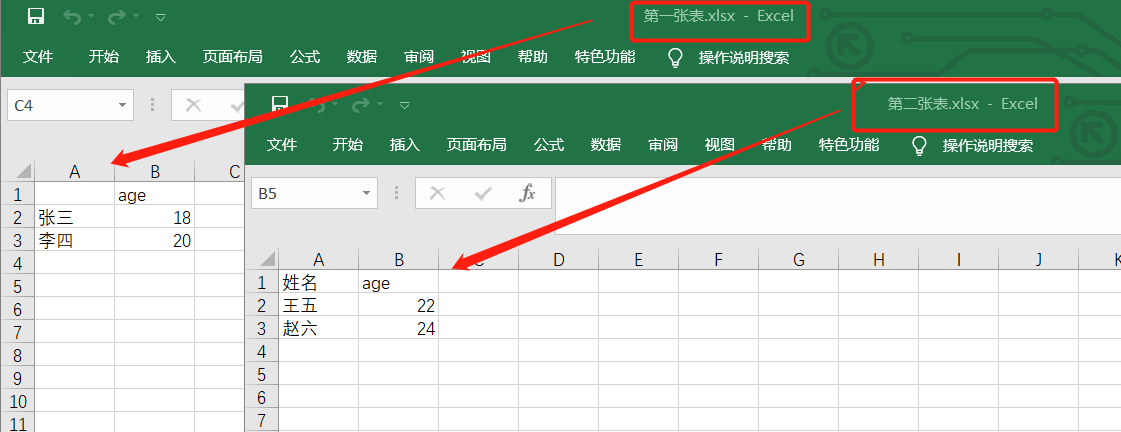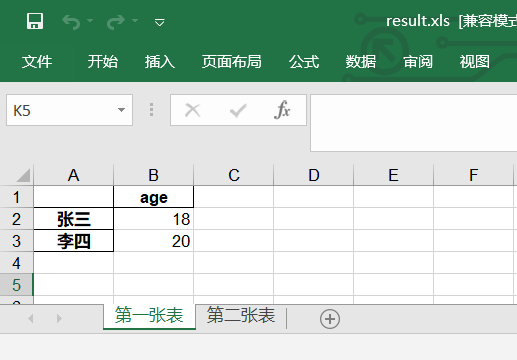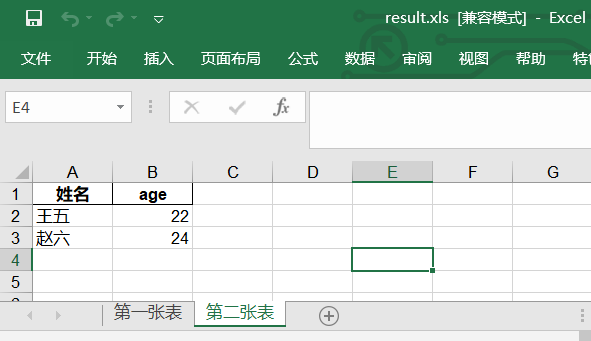展开全文数据挖掘
• 主要为大家详细介绍了Python多个excel文件合并为一个文件，具有一定的参考价值，感兴趣的小伙伴们可以参考一下
• 主要介绍了使用 Python 合并多个格式一致的 Excel 文件,本文给大家介绍的非常详细，具有一定的参考借鉴价值，需要的朋友可以参考下
• 这里需要用到python处理excel很经典的库openpyxl，安装也特别简单。window直接pip install就好了 ... 您可能感兴趣的文章:python实现跨excel的工作表sheet之间的复制方法python 实现读取一个excel多个shee
• 自打Python环境准备好之后，就在跟着网上的菜鸟教程学习基本操作、基本概念...此时，正巧碰到同事求助，他的第一个困惑是：需要在一个Excel中建若干个Sheet页，并且Sheet名需改为约定的名称。什么？这还不简单，打开...
• Python实现多个Sheet文件合并，添加列 需求：除了患者信息表，其它表添加patient_id字段； 代码如下 import os import pandas as pd ''' 需求：除了患者信息表，其它表添加patient_id字段； ''' base_dire = "./...
• 目录 split()截取一部分的字符串 读取文件夹下的所有Excel 模糊匹配符合条件的Excel split()截取一部分的字符串 str = 'https://www.baidu.com/pdf/abcdefg.pdf' # 输出字符串，>...# 做为一整体截...split 合并
• 读取一EXCEL所有的sheet数据 次运行追加写入csv文件 # -*- coding: utf-8 -*- """ Created on 2021/12/29 19:20 @author: tangge """ import pandas as pd import time path = "D:/code_fileAll/test_file/...
• 应懒癌朋友的要求，在这整理一下之前发过的几篇关于 excel 表格处理以及 pdf 文件相关的文章，如有需要自取哈～excel 表格处理相关： 用 python 整理 excel 表格 摘要：将一份表格文件中不同 sheet 单页面中的数据...
• 在生活中,我们经常会遇到这样的情况,即多个excel表格聚合为一种形式。例如,你分发表单班上所有学生填写,你负责结合成一个每个人的结果。有很多这样的问题。除了手动将所有表的内容复制到汇总表,如何使用Python自动...
• 主要介绍了pandas将多个dataframe以多个sheet的形式保存到一个excel文件中，文中通过示例代码介绍的非常详细，对大家的学习或者工作具有一定的参考学习价值，需要的朋友们下面随着小编来一起学习学习吧
• 站长现在工作的地方，最近经常用到Excel导入数据，公司的网站是PHP...每个类型码有多个Excel表，靠手工来操作不是程序员该干的事情，于是我用起了不是很熟练的Python来解决。代码如下，希望给新手一个帮助。（其实我...
• 有个Excel，里面有多个sheet，现在需要把每个sheet中固定单元格的数据提取出来 例如我的这个表格，每个月都有一个这样的表格，然后一共12个sheet存放了12个月的数据，现在我把每个sheet的C5单元格数据提取出来 ...excel
• Python数据分析】利用Python多个EXCEL表格合并为一个EXCEL表格。想获取代码文件，请微信关注微信公众号“闲谈项目管理”，对话框回复“数据分析案例2”即可获得文件下载链接。
• python 实现读取一个excel多个sheet表并合并的方法如下所示：import xlrdimport pandas as pdfrom pandas import DataFrameDATA_DIR = 'E:/'excel_name = '%s2017.xls' % DATA_DIRwb = xlrd.open_workbook(excel_...
• python 合并多个表格 版本：python 3.7 xlrd需要安装1.2版本 import xlrd,xlsxwriter,glob import os biao_tou = "NULL" wei_zhi = "NULL" # 获取要合并的所有excel表格 def get_exce(): global wei_zhi wei_zhi...
• Python实现Excel、多Sheet的合并处理。 三、项目准备 软件：PyCharm 需要的库：pandas， xlrd，os 四、项目分析 1）如何选择要合并的Excel文件？ 利用os，获取所有要合并的Excel文件。 2）如何选择要合并...pycharm 开发语言
• EXCEL的数值排序功能还是挺强大的，升序、降序，尤其自定义排序，能够对多个字段进行排序工作。 那么，在Python大法中，有没有这样强大的排序功能呢？答案是有的，而且本人觉得Python的排序功能，一点不比EXCEL的差...
• Python CSV 合并到多个sheet工作表 2、csv合并方法 import pandas as pd analysis_file = outDir+delimiter+'analysis_result.xlsx' writer = pd.ExcelWriter(analysis_file) csv_file1 = pd.read_csv...excel 合并...